# Test-Driven Development Tutorial using Visual Studio Unit Tests

By David Simmons January 21, 2016

Test-driven development (TDD) is a concept that I have started utilizing when writing code. If you are unfamiliar with this concept, TDD can simply be described as the practice of developing the tests before coding the actual logic. If you are new to this concept, it may seem backwards to write a test before having any code to run it against, but I have found that writing code this way can help eliminate many potential bugs in your code. In this blog post I will describe the basic process of developing code using TDD, and walk through an example of writing code using this strategy to demonstrate how you can use TDD to write code more effectively. (Note: Mocks, Fakes, Dependency Injection and Inversion of Control will not be discussed since the objective of this post is only to demonstrate the TDD process.)

When coding, many developers follow a process of first building out the logic, and then after it is completed, create a test to see if they get the expected results. There is nothing wrong with this approach and it will work for a lot of scenarios, but if you are working on a complex project that can have lots of varying inputs with many different algorithms to run, you may find yourself back tracking a lot to fix bugs. For example, if adding a new feature breaks existing code, this will result in extra time and cost if a good standard for testing is not used when developing the code.

With TDD, you would use the process of Red/Green/Refactor to write your code. The first step (Red) indicates that you will create a test to fail initially. The reason for this is to make sure there are no false positives in your code that would cause the test to pass. The next step (Green) is to write the minimum logic required for your test to pass. By only writing the minimal logic for the test, it helps you focus on getting an individual feature working before moving on to the next. Refactoring is then used to improve your code design while ensuring that all tests still pass. Since you are only writing the minimal amount of code needed at a time, you may find that certain elements can be written more efficiently as you continue adding more code. You will then repeat this cycle until all features of your code are implemented.

To go over this process in more detail, I will walk through developing code to calculate the area of an object given the object shape and parameters of that object. For this example, I write code to calculate the area of either a circle, rectangle, or triangle. To demonstrate the code for this example, I will be using Microsoft Visual Studio 2015 Community with C# as my language of choice.

To set this up in Visual Studio, I created a class library project called “CalculateArea” with an empty class called “AreaCalculatorClass” which will be used for calculating the area of an object.

Next, I created a new unit test project called “AreaTest” and I renamed the default test class accordingly. Below is what the solution should look like in the Solution Explorer: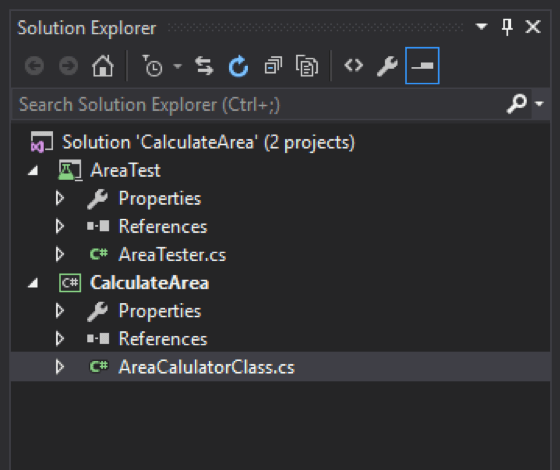Inside the AreaTest project, a reference will need to be added for the CalculateArea project, and a using directive for the CalculateArea namespace will need to be added to the AreaTester.cs code so that the tests can call the method that will be created in the AreaCalculatorClass. A new instance of AreaCalculatorClass will also need to be initialized inside the test class. Below is an example of what the code for that should look like in AreaTester.cs:

```using System;
using Microsoft.VisualStudio.TestTools.UnitTesting;
using CalculateArea;

namespace AreaTest
{
[TestClass]
public class AreaTester
{
private AreaCalculatorClass _target;

[TestInitialize]
public void Initialize()
{
_target = new AreaCalculatorClass();
}
[TestMethod]
public void TestMethod1()
{
}
}
}```

The code under [TestInitialize] will be ran before each test method, creating a new instance of the _target variable for each. This variable will be used to call the methods inside the AreaCalculatorClass.

For the first test, you will want to start off with something simple, and then through the TDD, test-driven development process, create additional tests. In this example, a good first test would be to test if valid parameters are used. For simplicity, I will assume the shape is a string and the parameters are in an integer array. Since there are three shapes to calculate the area of, this can be split into separate tests. To start, I will do tests for a circle.

To calculate the area of a circle, only one parameter is needed (radius) so the parameter array should only have one value when the shape is a circle. First, I renamed the default test method to “GivenCircleAndParameterLengthNotOneThrowException” (Note: If you are new to testing, it is always good to be as detailed as possible when naming your test methods so you know exactly what is being tested). As the name suggest for this test, I will use a circle with a parameter array length not equal to one, and expect and exception to be thrown. Specifically, I will use an ArgumentException and have the test method recognize this by adding an ExpectedException attribute above [TestMethod]:

[ExpectedException(typeof(ArgumentException))]

Now inside the test method, I will create the variables needed and call the area calculator method that does not exist yet.

```[ExpectedException(typeof(ArgumentException))]
[TestMethod]
public void WhenCircleShouldOnlyHaveOneParameter()
{
string shape = "circle";
int[] parameters = new int;
var result = _target.AreaCalculator(shape, parameters);
}```

I named the method AreaCalculator and called it with the shape and the integer array of length 0. Also I know the method will need to return a result for the area so I set a variable to store the result (although I am not concerned with that for this test). Since the AreaCalculator method does not exist in the AreaCalculatorClass yet, it will need to be created for the code to compile and run the test. In Visual Studio 2015, the new live code analysis feature will allow you to automatically generate this method. If you are using an older version, you can just create it manually: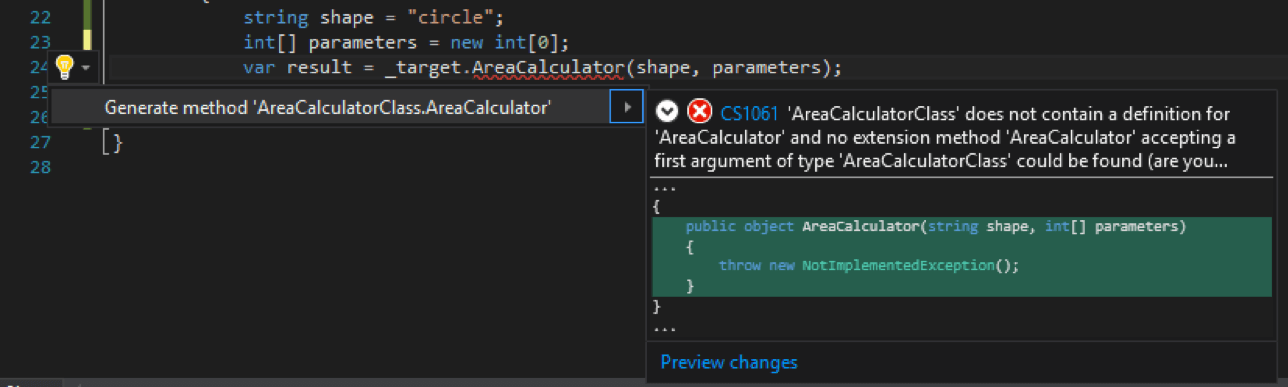Inside the AreaCalculatorClass.cs code, you can see the newly created method.

```public class AreaCalculatorClass
{
public object AreaCalculator(string shape, int[] parameters)
{
throw new NotImplementedException();
}
}```

For TDD, this works for the first step (Red) in the Red/Green/Refactor process since the test should fail with a different exception than expected. The solution can be built under Build->Build Solution, and the test ran from the test explorer under Test->Windows->Test Explorer.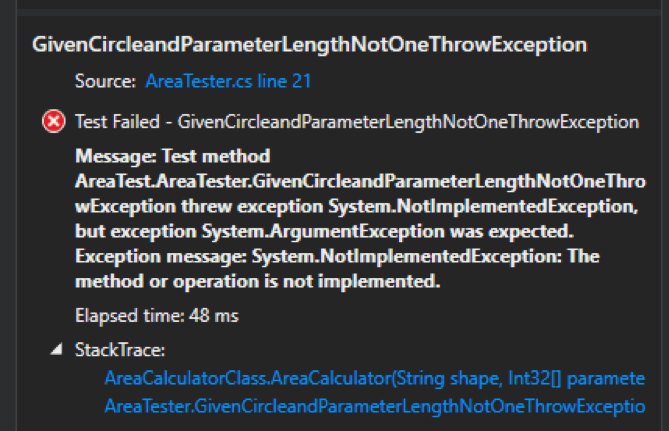As expected, the test fails. Now for the Green step, the minimum amount of code will need to be written for the test to pass (in this case, a pass will result in an ArgumentException). To do this, a simple if statement can be used to check if the parameters are correct.

```public object AreaCalculator(string shape, int[] parameters)
{
if (shape == "circle" && parameters.Length != 1)
{
throw new ArgumentException("Invalid Parameters for circle. Expected 1 value");
}
throw new NotImplementedException();
}```

What the code is doing now is simply checking that if the shape is a circle and the length or the parameter array is not one, throw an ArgumentException, otherwise the NotImplementedException will be thrown. Rebuilding and running the test should now result in a pass since the expected exception should be thrown.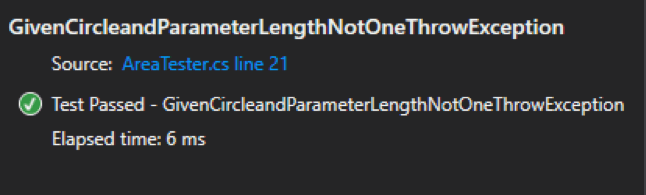Since this is the first test, there is not any refactoring that needs to be done yet, so I will demonstrate that with the next test.

Now that the logic is implemented for when incorrect circle parameters are used, the next test will be to test with correct circle parameters. For this test, the previous test method can be copy/pasted with just a few minor changes:

```[TestMethod]
public void GivenCircleAndParameterLengthIsOneShouldPass()
{
string shape = "circle";
int[] parameters = new int {1};
var result = _target.AreaCalculator(shape, parameters);
}```

The ExpectedException attribute is no longer needed since you should not get one for this test. I also changed the name of the test method and set the parameter array to a length of 1 with a single value. Since AreaCalculator will only throw an exception at this point, the test will fail (completing the Red step of the TDD process). You can recompile then run this new test to verify: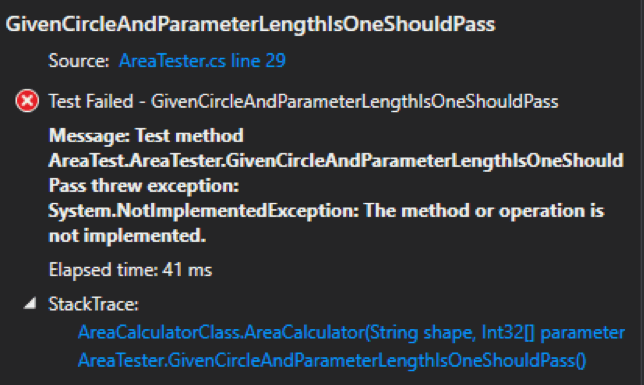To pass this test (thus satisfying the Green step), logic will need to be added to handle the valid parameters. To do this, I just added another if statement:

```public object AreaCalculator(string shape, int[] parameters)
{
if (shape == "circle" && parameters.Length != 1)
{
throw new ArgumentException("Invalid Parameters for circle. Expected 1 value");
}
if (shape == "circle" && parameters.Length == 1)
{
return null;
}
throw new NotImplementedException();
}```

Rerunning both test should now result in a pass.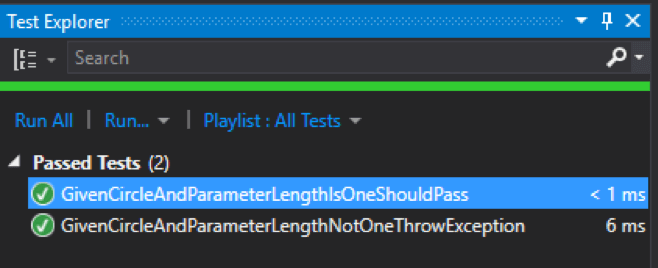Now that both test pass, the code can be refactored to improve the design. In this example, instead of using multiple if statements, this logic can be handled using a switch.  Below is an example of the improved design:

```public object AreaCalculator(string shape, int[] parameters)
{
switch (shape)
{
case "circle":
if (parameters.Length != 1)
{
throw new ArgumentException("Invalid Parameters for circle. Expected 1 value");
}
return null;
default:
throw new NotImplementedException();
}
}```

This design will allow us the easily add the logic for the other shapes and removes unnecessary if statements.

After refactoring, all test should be rerun to ensure they still pass. In this case, both test still pass so the next tests can be created.

Since the parameter tests for rectangle and triangle will be very similar, the circle tests can be copy/pasted and modified accordingly to save time. The resulting tests are below:

```[ExpectedException(typeof(ArgumentException))]
[TestMethod]
public void GivenRectangleAndParameterLengthNotTwoThrowException()
{
string shape = "rectangle";
int[] parameters = new int;
var result = _target.AreaCalculator(shape, parameters);
}

[TestMethod]
public void GivenRectangleAndParameterLengthIsTwoShouldPass()
{
string shape = "rectangle";
int[] parameters = new int {1,2};
var result = _target.AreaCalculator(shape, parameters);
}

[ExpectedException(typeof(ArgumentException))]
[TestMethod]
public void GivenTriangleAndParameterLengthNotTwoThrowException()
{
string shape = "triangle";
int[] parameters = new int;
var result = _target.AreaCalculator(shape, parameters);
}

[TestMethod]
public void GivenTriangleAndParameterLengthIsTwoShouldPass()
{
string shape = "triangle";
int[] parameters = new int {1,2};
var result = _target.AreaCalculator(shape, parameters);
}```

The red/green/refactor process can now be used for each of these test individually. Starting with GivenRectangleAndParameterLengthNotTwoThrowException, running the test should fail. To pass this test, all that is needed in our logic is a case for “rectangle” and to throw an exception for invalid parameter length:

```switch (shape)
{
case "circle":
if (parameters.Length != 1)
{
throw new ArgumentException("Invalid Parameters for circle. Expected 1 value");
}
return null;
case "rectangle":
if (parameters.Length != 2)
{
throw new ArgumentException("Invalid Parameters for rectangle. Expected 2 values");
}
throw new NotImplementedException();
default:
throw new NotImplementedException();
}```

Again, I used NotImplementedException as a placeholder for logic that is not yet implemented to ensure that there are no false positives for the other tests. Running all test should only result in the GivenRectangleAndParameterLengthNotTwoThrowException and the previous two tests to pass.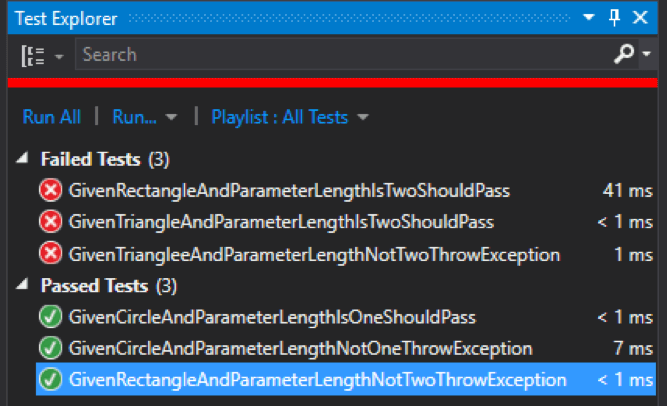Repeating the red/green/refactor process for the remaining tests should result in a case for each shape and passing tests: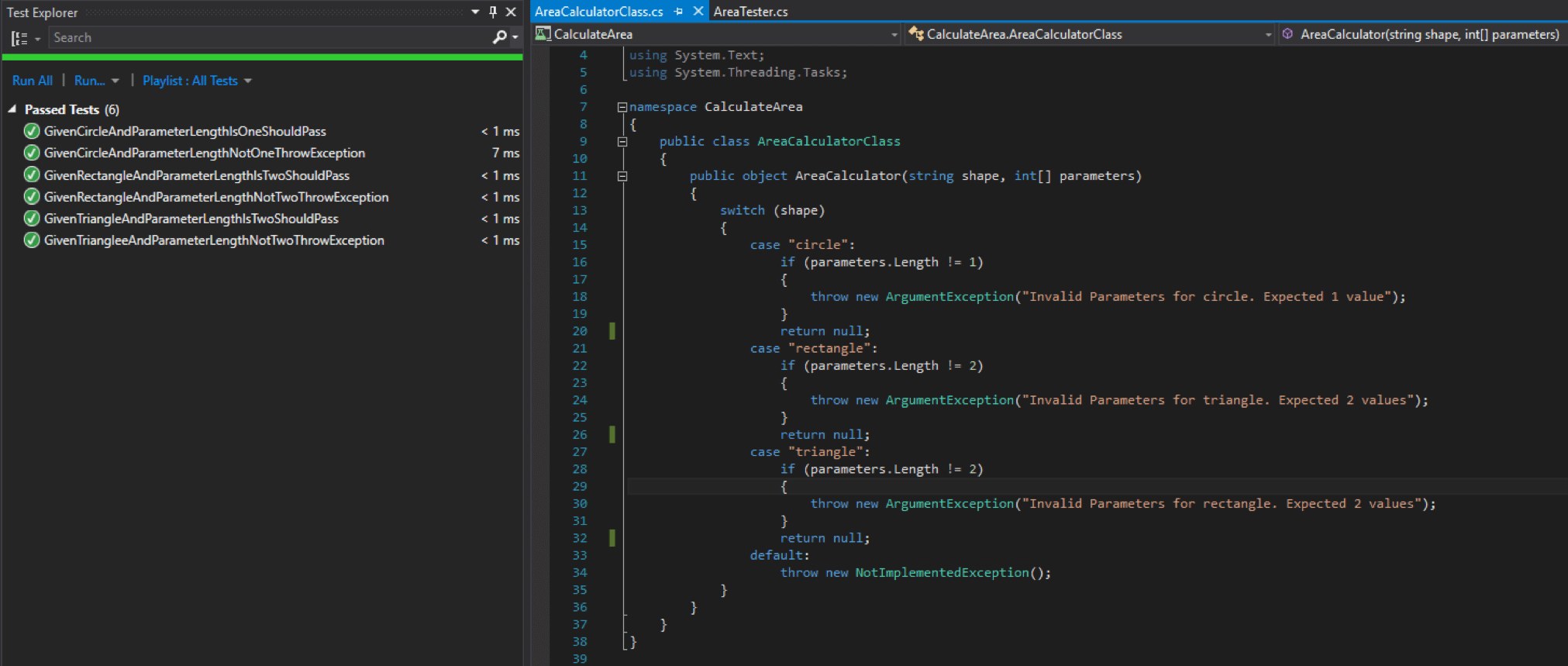Now that the logic to handle correct and incorrect parameters is there, all that is left to test is the calculation of the areas for each shape. Starting with circle, the test should be created to check the expected result based on the parameters. The code for GivenCircleAndParameterLengthIsOneShouldPass can be copied to a new test method with just a few minor changes:

```[TestMethod]
public void GivenCircleAndParameterOfTwoAreaShouldEqual12_57()
{
string shape = "circle";
int[] parameters = new int {2};
var result = _target.AreaCalculator(shape, parameters);
Assert.AreEqual(12.57, result, "Test failed: improper calculation.");
}```

For this test, we will expect a radius of 2 to result in an area of 12.75. Running this test with our current logic will fail since a null value is being returned.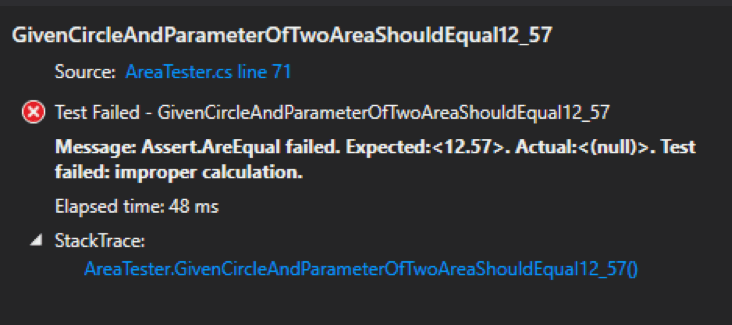To resolve this, instead of returning null, that can be replaced with the formula for calculating the area.

```case "circle":
if (parameters.Length != 1)
{
throw new ArgumentException("Invalid Parameters for circle. Expected 1 value");
}
return Math.Round(Math.PI*Math.Pow(parameters,2),2);```

For this example, it needed to round to two decimal places to match the expected results. So running the tests should now result in a pass. Repeating this process for the rectangle and triangle should result in test similar to below:

```[TestMethod]
public void GivenRectangleAndParameterOf2x4AreaShouldEqual8()
{
string shape = "rectangle";
int[] parameters = new int {2,4};
var result = _target.AreaCalculator(shape, parameters);
Assert.AreEqual(8, result, "Test failed: improper calculation.");
}

[TestMethod]
public void GivenTriangleAndParameterOf2x4AreaShouldEqual4()
{
string shape = "triangle";
int[] parameters = new int {2,4};
var result = _target.AreaCalculator(shape, parameters);
Assert.AreEqual(4.0, result, "Test failed: improper calculation.");
}
And the following code in the area calculator:
case "rectangle":
if (parameters.Length != 2)
{
throw new ArgumentException("Invalid Parameters for rectangle. Expected 2 values");
}
return parameters*parameters;
case "triangle":
if (parameters.Length != 2)
{
throw new ArgumentException("Invalid Parameters for rectangle. Expected 2 values");
}
return 0.5*parameters*parameters;```

Note that a decimal is used in the triangle test code so that it forces a “double” type to match the result.

Now that all test should pass, any final refactoring can be done to the code. For advanced developers, this code can be refactored to follow the SOLID Open/closed principle, but that is a blog post for another day.

The most important concept to take away from using test-driven development is how it makes you think about your code. Through the Red/Green/Refactor process, you must think about how each individual feature in your code must function, make it work, and finally make it work efficiently.

I hope you found this tutorial helpful and can refer to this for how you can use TDD in your projects. Be sure to visit our website to learn more about our Application Development practice at ivision, and fill out our contact form if you would like to keep in touch.